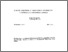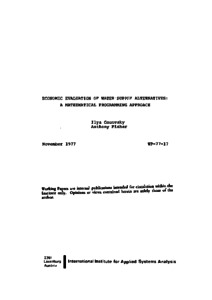# Economic Evaluation of Water Supply Alternatives: A Mathematical Programming Approach

Gouevsky, I.V. & Fisher, A.C. (1977). Economic Evaluation of Water Supply Alternatives: A Mathematical Programming Approach. IIASA Working Paper. IIASA, Laxenburg, Austria: WP-77-017Preview
Text
WP-77-017.pdf

## Abstract

The main task of this paper is to propose a method for deriving regional water supply functions, taking into account a variety of supply alternatives and some engineering and environmental aspects of each. The purpose is to provide a framework for decisions about the efficient use of a region's water resources. The first section deals with distinctions between engineering and economics. The notion of supply-demand equilibrium and the economic efficiency properties of this equilibrium are reviewed. The second section surveys the "State-of-the-Art" in regional water supply, describing a number of alternative sources of supply. The third section considers how, for a region having just two inputs, each point on a supply curve can be derived as the solution to a nonlinear program to minimize the cost of obtaining a given quantity of water. The procedure is however perfectly general, and in the fourth section an application is made to a hypothetical region with several sources of supply, each having several inputs, with constraints on their use, and so on. An interesting feature of the model is that it can -- and does, in the application -- reflect environmental constraints as well. For ease in computation the production relations are linearized in order to use a linear programming solution algorithm. Based on the assumed production relations and resource constraints, a well behaved regional water supply function is derived.

Item Type: Monograph (IIASA Working Paper) Resources and Environment Area (REN) IIASA Import 15 Jan 2016 01:44 27 Aug 2021 17:08 http://pure.iiasa.ac.at/717View Item